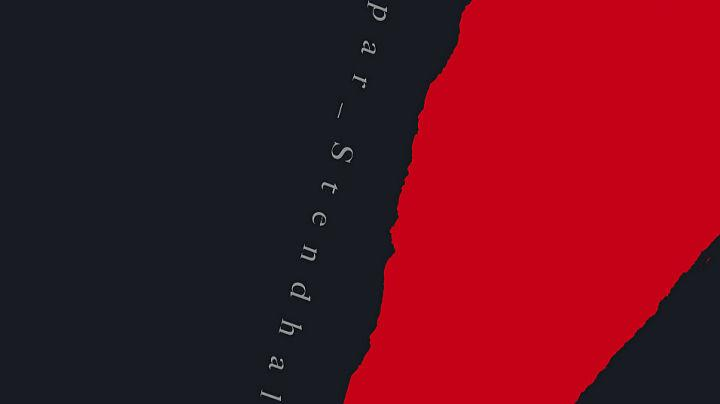## ​前言

1. 为什么会有红黑树？
2. ​红黑树的应用场景和定义？
3. 红黑树的高效和稳定是怎么实现？

### 为什么会有红黑树

• 红黑树可以保证 最好 最坏 情况的所有操作（插入/删除/查找等）时间复杂度都是对数级别 O(logN)
• 和二叉树不同，无论插入顺序如何，红黑树都是接近完美平衡的
• 无数实验的应用证明，红黑树的操作成本（包括旋转和变色）比二叉树降低40%左右

### 红黑树的应用场景和定义

• Java 的 HashMap （8 以后）的链表树化是通过 红黑树实现
• Java 的 TreeMap 是通过红黑树实现
• Nginx 是用红黑树管理 timer 等
• Linux 进程调度用红黑树管理进程控制块
• 等等……

• 红链接必须为左链接
• 不能出现两条相连的红链接
• 该树是完美黑色平衡的

``````    private Node put(Node h, Key key, Value val) {
// 二分插入
if(h == null) return new Node(key, val, RED, 1);
int cmp = key.compareTo(h.key);
if(cmp < 0) h.left = put(h.left, key, val);
else if(cmp > 0) h.right = put(h.right, key, val);
else h.val = val;

// 修复 右倾连接
if(isRed(h.right) && !isRed(h.left)) h = rotateLeft(h);         // 违反规则 不允许出现左右红连接
if(isRed(h.left) && isRed(h.left.left)) h = rotateRight(h);     // 违反规则 不允许出现连续的左红连接
if(isRed(h.left) && isRed(h.right)) flipColors(h);              // 当左右子节点为红色， 则全部变色
h.size = size(h.left) + size(h.right) + 1;
return h;
}``````

### 红黑树的高效和稳定是怎么实现？

• 左旋转（当出现右红子节点时，进行左旋转）
• 右旋转（当出现两条相连的左子红链接时，进行右旋转）
• 变色（当左右节点都是红链接时，进行变色）

``````    private Node rotateLeft(Node h) {
Node x = h.right;
h.right = x.left;
x.left = h;
x.color = x.left.color;
x.left.color = RED;
x.size = h.size;
h.size = size(h.left) + size(h.right) + 1;
return x;
}
``````

``````    private Node rotateRight(Node h) {
Node x = h.left;
h.left = x.right;
x.right = h;
x.color = x.right.color;
x.right.color = RED;
x.size = h.size;
h.size = size(h.left) + size(h.right) + 1;
return x;
}
``````

``````    private void flipColors(Node h) {
h.color = !h.color;
h.left.color = !h.left.color;
h.right.color = !h.right.color;
}``````

1. A首先成为根节点
2. C首先插入在A的右边，A违反了不能出现红右子节点的规则，进行左旋转，A成了C的左红子节点
3. E首先插入在C的右边，C违反左右子节点均为红色的规则，进行变色，C,A,E变黑（根节点永远为黑）
4. H首先插入在E的右边，E违反了不能出现红右子节点的规则，进行左旋转，E成了H的左红子节点
5. L首先插入在H的右边，H违反左右子节点均为红色的规则，进行变色，E,L变黑，H变红，导致C违反了不能出现红右子节点的规则，进行左旋转，C成为H的左红子节点（这里违反2个规则）
6. M首先插入在L的右边，L违反了不能出现红右子节点的规则，进行左旋转，L成为M的左红子节点
7. P首先插入在M的右边，M违反左右子节点均为红色的规则，进行变色，L,P变黑，M变红，导致H违反左右子节点均为红色的规则，进行变色，H,C,M变黑（这里违反2个规则）
8. R首先插入到P的右边，P违反了不能出现红右子节点的规则，进行左旋转，P成为R的左红子节点
9. S首先插入到R的右边，R违反左右子节点均为红色的规则，进行变色，S,P变黑，R变红，导致M违反了不能出现红右子节点的规则，进行左旋转，M成为R的左红子节点
10. X首先插入到S的右边，S违反了不能出现红右子节点的规则，进行左旋转，S成为X的左红子节点

### 总结

algs4.cs.princeton.edu/

algs4.cs.princeton.edu/

zh.wikipedia.org/wiki/%